# US5042927A - Compact zoom lens - Google Patents

Compact zoom lens Download PDF

## Info

Publication number
US5042927A
US5042927A US07/449,710 US44971089A US5042927A US 5042927 A US5042927 A US 5042927A US 44971089 A US44971089 A US 44971089A US 5042927 A US5042927 A US 5042927A
Authority
US
United States
Prior art keywords
lens
wide
angle
groups
telephoto
Prior art date
Legal status (The legal status is an assumption and is not a legal conclusion. Google has not performed a legal analysis and makes no representation as to the accuracy of the status listed.)
Expired - Lifetime
Application number
US07/449,710
Inventor
Hideki Ogawa
Hiroshi Endo
Current Assignee (The listed assignees may be inaccurate. Google has not performed a legal analysis and makes no representation or warranty as to the accuracy of the list.)
Canon Inc
Original Assignee
Canon Inc
Priority date (The priority date is an assumption and is not a legal conclusion. Google has not performed a legal analysis and makes no representation as to the accuracy of the date listed.)
Filing date
Publication date
Priority to JP63323045A priority Critical patent/JP2605386B2/en
Priority to JP63-323045 priority
Priority to JP32438988A priority patent/JP2576609B2/en
Priority to JP63-324388 priority
Priority to JP63-324389 priority
Priority to JP63324388A priority patent/JP2560815B2/en
Application filed by Canon Inc filed Critical Canon Inc
Assigned to CANON KABUSHIKI KAISHA, A CORP. OF JAPAN reassignment CANON KABUSHIKI KAISHA, A CORP. OF JAPAN ASSIGNMENT OF ASSIGNORS INTEREST. Assignors: ENDO, HIROSHI, OGAWA, HIDEKI
Application granted granted Critical
Publication of US5042927A publication Critical patent/US5042927A/en
Anticipated expiration legal-status Critical
Expired - Lifetime legal-status Critical Current

## Links

• 238000000926 separation method Methods 0.000 claims abstract description 10
• 230000003247 decreasing Effects 0.000 claims abstract 3
• 230000004075 alteration Effects 0.000 description 14
• 230000003287 optical Effects 0.000 description 6
• 238000004904 shortening Methods 0.000 description 5
• 241000226585 Antennaria plantaginifolia Species 0.000 description 2
• 230000003595 spectral Effects 0.000 description 2
• 238000010586 diagram Methods 0.000 description 1
• 230000000694 effects Effects 0.000 description 1
• 239000011521 glass Substances 0.000 description 1
• 230000000717 retained Effects 0.000 description 1
• 230000000087 stabilizing Effects 0.000 description 1
• 230000001629 suppression Effects 0.000 description 1

## Images

•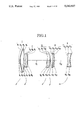•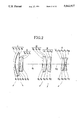•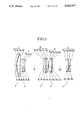•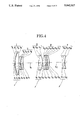•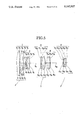•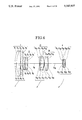•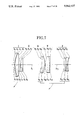•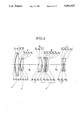•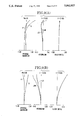•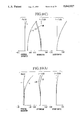•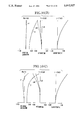•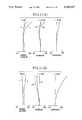•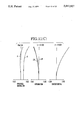•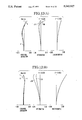•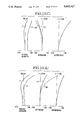•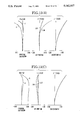•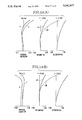•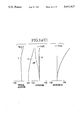•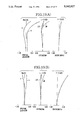•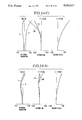•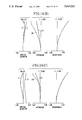## Classifications

• GPHYSICS
• G02OPTICS
• G02BOPTICAL ELEMENTS, SYSTEMS, OR APPARATUS
• G02B15/00Optical objectives with means for varying the magnification
• G02B15/14Optical objectives with means for varying the magnification by axial movement of one or more lenses or groups of lenses relative to the image plane for continuously varying the equivalent focal length of the objective
• G02B15/145Optical objectives with means for varying the magnification by axial movement of one or more lenses or groups of lenses relative to the image plane for continuously varying the equivalent focal length of the objective having five groups only
• G02B15/1451Optical objectives with means for varying the magnification by axial movement of one or more lenses or groups of lenses relative to the image plane for continuously varying the equivalent focal length of the objective having five groups only the first group being positive
• G02B15/145113Optical objectives with means for varying the magnification by axial movement of one or more lenses or groups of lenses relative to the image plane for continuously varying the equivalent focal length of the objective having five groups only the first group being positive arranged +-++-
• GPHYSICS
• G02OPTICS
• G02BOPTICAL ELEMENTS, SYSTEMS, OR APPARATUS
• G02B15/00Optical objectives with means for varying the magnification
• G02B15/14Optical objectives with means for varying the magnification by axial movement of one or more lenses or groups of lenses relative to the image plane for continuously varying the equivalent focal length of the objective
• G02B15/16Optical objectives with means for varying the magnification by axial movement of one or more lenses or groups of lenses relative to the image plane for continuously varying the equivalent focal length of the objective with interdependent non-linearly related movements between one lens or lens group, and another lens or lens group
• G02B15/163Optical objectives with means for varying the magnification by axial movement of one or more lenses or groups of lenses relative to the image plane for continuously varying the equivalent focal length of the objective with interdependent non-linearly related movements between one lens or lens group, and another lens or lens group having a first movable lens or lens group and a second movable lens or lens group, both in front of a fixed lens or lens group
• G02B15/167Optical objectives with means for varying the magnification by axial movement of one or more lenses or groups of lenses relative to the image plane for continuously varying the equivalent focal length of the objective with interdependent non-linearly related movements between one lens or lens group, and another lens or lens group having a first movable lens or lens group and a second movable lens or lens group, both in front of a fixed lens or lens group having an additional fixed front lens or group of lenses
• G02B15/173Optical objectives with means for varying the magnification by axial movement of one or more lenses or groups of lenses relative to the image plane for continuously varying the equivalent focal length of the objective with interdependent non-linearly related movements between one lens or lens group, and another lens or lens group having a first movable lens or lens group and a second movable lens or lens group, both in front of a fixed lens or lens group having an additional fixed front lens or group of lenses arranged +-+

## Abstract

A zoom lens is disclosed comprising, from front to rear, a first lens group of positive power, a second lens group of negative power, a third lens group of positive power, a fourth lens group of positive power, and a fifth lens group of negative power, at least a predetermined plurality of lens groups of the first to fifth lens groups being made to move in such a manner that when zooming from the wide-angle end to the telephoto end, the first lens group is moved forward, while simultaneously monotonously increasing the air separation between the first and second lens groups and monotonously decreasing the air separations between the second and third lens groups and between the fourth and fifth lens groups, and the zoom lens satisfying the following conditions: 0.05 fT/fW<Δe34/DW<0.15 fT/fW 0.05<f5/f12W<0.6 where fW and fT are the shortest and longest focal lengths of the entire lens system, f5 is the focal length of the fifth lens group, f12W is the overall focal length of the first and second lens groups at the wide-angle end, Δe34 is a changed amount of the principle point interval between the third and fourth lens groups at the telephoto end from that at the wide-angle end and DW is the length of the entire lens system from the first lens surface to the last lens surface.

## Description

BACKGROUND OF THE INVENTION
1. Field of the Invention
This invention relates to compact zoom lenses of the telephoto type including long focal lengths suited to 35 m/m cameras, video cameras and electronic still cameras and, more particularly, to compact zoom lenses having five lens groups, a plurality of lenses of which are made to move to vary the image magnification, so that the optical performance is kept high over the entire range of magnification of about 2.5.
2. Description of the Related Art
There are many previous proposals for a zoom lens having five or more lens groups, of which at least three lens groups are made to move for zooming purposes in order to achieve a minimization of the entire lens system while securing the predetermined zoom ratio.
Of these, ones which are comprised entirely of five lens groups and have three or more of their lens groups made to move when varying the image magnification are proposed, for example, in Japanese Laid-Open Patent Application No. Sho 60-175020 and Japanese Laid-Open Patent Application No. Sho 63-189819.
The Japanese Laid-Open Patent Application No. Sho 60-1755020 is proposing zoom lenses of the telephoto type including relatively long focal lengths. In this document, either all of the five lens groups having predetermined refractive powers are made to move, or four of them, say, the first, third, fourth and fifth lens groups, are made to move toward, for example, the object side, when the image magnification is varied from the wide-angle end to the telephoto end, thereby providing a range of 3 and a minimum F-number in about 3.6 to 5.6.
Another document or the Japanese Laid-Open Patent Application No. Sho 63-189819 is proposing zoom lenses of the standard form including relatively wide angles of view, when zooming from the wide-angle end to the telephoto end, of the five lens groups having predetermined refractive powers, the first lens group is made to move toward the object side, while the third, fourth and fifth lens groups are made to move in either fixedly or variably spaced relation, as a whole, toward the object side.
In such a way, the zoom lens may entirely be constructed from five lens groups so that a plurality of not less than three of these lens groups can be arranged to move, while maintaining predetermined relations to each other, in order to vary the image magnification. Because this zoom type facilitates a minimization of the size of the entirety of the lens system while securing the zoom ratio at a certain value, it has found its use in the zoom lenses of 35 m/m cameras, video cameras, etc..
With such a zoom type in use, when achieving a further minimization of the bulk and size of the whole lens system by shortening its total length, it is usual to take either of the measures that the number of lens members in each group is reduced, and that the refractive power of each lens group is strengthened.
Relying merely on either or both of the reduction of the number of lens members and the increase of the refractive power of each lens group, however, leads to an insufficiency of aberration correction in each lens group and also an increase of the range of variation of aberrations with variation of the image magnification, thus giving a problem that it becomes very difficult to obtain high grade imagery over the entire range of variation of the image magnification.
SUMMARY OF THE INVENTION
An object of the invention is to provide a compact zoom lens as constructed entirely from five lens groups having predetermined refractive powers, wherein proper ranges for the refractive powers of all the lens groups and proper conditions for the movements of all the zooming lens groups are set forth to achieve a much desired shortening of the total length of the entire system in such a manner that a high optical performance is maintained throughout the entire range of variation of magnification.
To accomplish such an object, according to the invention, the zoom lens has, in the order from the front, a first lens group of positive refractive power, a second lens group of negative refractive power, a third lens group of positive refractive power, a fourth lens group of positive refractive power and a fifth lens group of negative refractive power, at least a predetermined plurality of lens groups of the first, second, third, fourth and fifth lens groups being made to move in such a manner that when zooming from the wide angle-end to the telephoto end, the first lens group is moved forward, while simultaneously the air separation between the first lens group and the second lens group monotonously increases, the air separation between the second lens group and the third lens group monotonously decreases, and the air separation between the fourth lens group and the fifth lens group monotonously decreases. And, the invention has set forth inequalities of conditions to be described later.
BRIEF DESCRIPTION OF THE DRAWINGS
FIG. 1 to FIG. 8 are longitudinal section views of examples 1 to 8 of specific zoom lenses of the invention respectively.
FIGS. 9(A) to 9(C), 10(A) to 10(C), 11(A) to 11(C), 12(A) to 12(C), 13(A) to 13(C), 14(A) to 14(C), 15(A) to 15(C) and 16(A) to 16(C) are graphic representations of the various aberrations of the zoom lenses of FIGS. 1 to 8 respectively. In these graphs, FIGS. 9(A), 10(A), 11(A), 12(A), 13(A), 14(A), 15(A) and 16(A) show the aberrations in the wide-angle end, FIGS. 9(B), 10(B), 11(B), 12(B), 13(B), 14(B), 15(B) and 16(B) in an intermediate position, and FIGS. 9(C), 10(C), 11(C), 12(C), 13(C), 14(C), 15(C) and 16(C) in the telephoto end.
In these drawings, I, II, III, IV and V denote consecutively the first, second, third, fourth and fifth lens groups; ΔS denotes the sagittal image surface and ΔM the meridional image surface; d denotes the spectral d-line, and g the spectral g-line; y denotes the image height; and the arrows indicate the loci of motion of the lens groups when zooming from the wide-angle end to the telephoto end.
DETAILED DESCRIPTION OF THE PREFERRED EMBODIMENTS
FIG. 1 to FIG. 8 in lens block diagrams show numerical examples 1 to 8 of the invention, wherein the zoom lenses each comprises a first lens group I of positive refractive power, a second lens group II of negative refractive power, a third lens group III of positive refractive power, a fourth lens group IV of positive refractive power and a fifth lens group V of negative refractive power. The arrows indicate the loci of motion of all or some of these lens groups when zooming from the wide-angle end to the telephoto end.
In the present embodiment, in order to achieve a minimization of the size of the entire lens system, the number of lens members in each lens group is made as few as possible. For this purpose, as shown in FIGS. 1 to 8, the entirety is constructed from five lens groups of predetermined refractive powers, and these lens groups are arranged upon zooming to move in such ways as shown in the drawings that they have their shares to a magnification varying effect made in good balance.
Another feature is that each lens group is made to move in such a way that, when zooming from the wide-angle end to the telephoto end, the air separation between the first lens group and the second lens group monotonously increases, the air separation between the second lens group and the third lens group monotonously decreases, and the air separation between the fourth lens group and the fifth lens group monotonously decreases.
In the present embodiment, for the numerical examples 1 and 3, as shown in FIGS. 1 and 3, the first lens group and the third lens group are moved forward, and the second lens group, the fourth lens group and the fifth lens group are moved so as to depict loci convex toward the rear, when zooming is performed from the wide-angle end to the telephoto end.
For the numerical example 2, as shown in FIG. 2, the first lens group, the third lens group and the fifth lens group are moved forward, the second lens group is moved rearward, and the fourth lens group is moved so as to depict a locus convex toward the rear, when zooming is performed from the wide-angle end to the telephoto end.
For the numerical examples 4 to 6, as shown in FIGS. 4 to 6, when zooming from the wide-angle end to the telephoto end, the second lens group and the fourth lens group are made to remain stationary, and the first, third and fifth lens groups are moved forward independently of each other.
For the numerical examples 7 and 8 shown in FIGS. 7 and 8, when zooming from the wide-angle end to the telephoto end, the first lens group is moved forward, while the third lens group and the fifth lens group are simultaneously moved forward in unified relation. Of these, in the numerical example 7 of FIG. 7, the second lens group and the fourth lens group are fixed, and in the numerical example 8 of FIG. 8, though the second lens group moves, the fourth lens group is fixed.
Further, the zoom lenses of the numerical examples 1 to 8 of the invention are made to satisfy the following conditions:
`0.15 fT/fW<Δe34/DW<0.15 fT/fW                        (1)`
`0.05<f5/f12W<0.6                                           (2)`
where fW and fT are focal lengths of the entire lens system at the wide-angle end and the telephoto end respectively, f5 is a focal length of the fifth lens group, f12W is an overall focal length of the first and second lens groups at the wide-angle end, Δe34 is a changed amount of the interval between principal points of the third and fourth lens groups at the telephoto end from that at the wide-angle end, and DW is the length of the entire lens system from the first lens surface to the last lens surface at the wide-angle end.
Thus, in the present embodiment, the minimization of the size of the entire lens system is achieved in such a manner that the back focal distance does not become unnecessarily long. For this purpose, the refractive powers and paraxial arrangement of all the lens groups are so determined that the entire lens system, when in the wide-angle end, does not get too strong in the inverted telephoto type, in other words, becomes to be of a relatively weak inverted telephoto type.
Next, the technical significance of each of the above-described conditions is explained.
The inequalities of condition (1) define a range for the amount of change of the interval between the principal points of the third and fourth lens groups when zooming from the wide-angle end to the telephoto end relative to the total length of the lens at the wide-angle end. When the principal point interval between the third and fourth lens groups becomes too long beyond the upper limit of the inequalities of condition (1), a necessity arises that the space for their zooming movement be secured so large that they do not interfere with each other in the wide-angle end. As a result, the total length of the lens in the wide-angle end increases objectionably.
Further, when the lower limit of the inequalities of condition (1) is exceeded, the principal point interval between the third and fourth lens groups becomes too short in the telephoto end. To fulfill both of the requirements of obtaining the desired zoom ratio and of shortening the optical total length in the wide-angle end, therefore, the zoom strokes of the first lens group and the fifth lens group must be increased. Therefore, this is not advantageous.
The inequalities of condition (2) define a range for the ratio of the focal length of the fifth lens group to the overall focal length of the first and second lens groups. When the negative refractive power of the fifth lens group becomes too weak beyond the upper limit of the inequalities of condition (2), the distortion tends to decrease, but a necessity arises that the spacing between the fourth and fifth lens groups in the wide-angle end be taken wide in order to obtain the desired zoom ratio. Further, when in the wide-angle end, the back focal distance becomes longer than necessary. Hence, it becomes difficult to achieve an advance in the compactness of the entire lens system.
Further, when the lower limit of the inequalities of condition (2) is exceeded, the negative refractive power of the fifth lens group becomes too strong to ease the difficulty of well correcting aberrations over the entire area of the image frame, since large pincushion distortion is produced and the Petzval sum increases in the negative direction. Therefore, it is not desirable.
To achieve a good correction of the variation of aberrations with zooming for good stability of optical performance throughout the entire zooming range, the invention now sets forth the following condition:
`-3.5<f12W/fW<-1.3                                          (3)`
The inequalities of condition (3) define a range for the ratio of the overall focal length of the first and second lens groups in the wide-angle end to the shortest focal length of the entire system. When this condition is satisfied, an improvement of the compact form of the entire lens system can be attained particularly with the back focal distance made to be of the optimum length, while still suppressing the variation of aberrations with zooming.
When the upper limit of the inequalities of condition (3) is exceeded, the overall negative refractive power of the first and second lens groups becomes too weak. This is advantageous at reduction of the outer diameters of the third lens group and those that follow, and, in a case where the diaphragm is positioned on the image side of the second lens group, at shortening of the outer diameter of the lens barrel owing to the reduction of the diameter of the diaphragm. But, the requirements of securing the necessary back focal distance and of minimizing the physical length of the entire lens system can hardly be fulfilled at once. It is also required to strengthen the negative refractive power of the fifth lens group. This results in increasing the pincushion distortion.
Further, when the overall negative refractive power of the first and second lens groups is too much strong as exceeding the lower limit of the inequalities of condition (3), the back focal distance becomes too long. Hence, the entire lens system is caused to increase in the longitudinal direction. And the various aberrations, particularly the spherical aberration and the curvature of field, vary to larger extent. To secure the necessary minimum F-number, the diameter of the diaphragm has to be increased. Thus, the lens barrel is increased also in the lateral direction. Therefore, it is not desirable.
To achieve a further minimization of the bulk and size of the entire lens system while still preserving high optical performance throughout the entire zooming range, the invention sets forth the following condition, too:
`0.35<f3/f4<2.5                                             (4)`
where f3 and f4 are focal lengths of the third and fourth lens groups respectively.
The inequalities of condition (4) define a range for the ratio of the focal length of the third lens group to the focal length of the fourth lens group. When the positive refractive power of the third lens group is too much weak as exceeding the upper limit of the condition (4), the spacing between the fourth and fifth lens groups in the wide-angle end has to be taken at a large value. Otherwise, the predetermined zoom ratio could not be obtained. Hence, it becomes difficult to attain an improved result of the compact form of the lens system. If the desired compactness of the lens system is retained, the refractive power of the second lens group or the fifth lens group must be strengthened. This results in an increase in the difficulty of well correcting aberrations.
Further, when the lower limit of the condition (4) is exceeded, the refractive power of the third lens group becomes too much strong. Therefore, the third lens group produces large aberrations. Particularly the spherical aberration gets larger, which is hardly corrected in good balance by the design of the other lens groups.
When all the foregoing conditions are satisfied, realization of a zoom lens the invention aims at can be achieved. Yet, to assist in simultaneous fulfillment of the requirements of achieving a much desired minimization of the bulk and size of the entire lens system and of stabilizing the aberrations against zooming for high optical performance, it is preferred in the present embodiment that the first lens group counting from the front is constructed from a meniscus-shaped negative lens convex toward the front and a positive lens, the second lens group is constructed from a cemented doublet consisting of a negative lens in bi-concave form and a positive lens, the third lens group is constructed with inclusion of a negative lens and at least one positive lens, the fourth lens group is constructed with inclusion of at least one positive lens and at least one negative lens, and the fifth lens group is constructed from at least one positive lens and at least one negative lens in bi-concave form.
Alternatively, it is preferred that the first lens group is constructed from a negative lens and at least one positive lens, the second lens group is constructed from a negative lens in bi-concave form and a positive lens, the third lens group is constructed with inclusion of a negative lens and a positive lens, the fourth lens group is constructed with inclusion of at least one positive lens and at least one negative lens, and the fifth lens group is constructed from at least one positive lens and at least one negative lens.
Further, in application of the invention to the case shown in FIGS. 4 to 6 where the second lens group and the fourth lens group are fixed, and, when zooming from the wide-angle end to the telephoto end, the first, third and fifth lens groups are moved forward according to respective different loci from each other, it is preferred to set forth the ranges for the factors of the before-described inequalities of conditions as follows: ##EQU1##
Meanwhile, in application to the case shown in FIGS. 7 and 8 where when zooming from the wide-angle end to the telephoto end, the fourth lens group remains stationary, the first lens group moves forward, and the third lens group and the fifth lens group move forward in unison, it is preferred to set forth the ranges for the factors of the before-described inequalities of conditions as follows: ##EQU2##
The examples 1 to 8 of specific zoom lenses of the invention can be constructed in accordance with the numerical data given in the tables below for the radii of curvature, R, the axial thicknesses or air separations, D, and the refractive indices, N, and the Abbe numbers, v, of the glasses of the lens elements with the subscripts numbered consecutively from front to rear.
The numerical values of the factors of the before-described inequalities of conditions (1) to (4) for the numerical examples 1 to 8 are listed in Table 1.
`______________________________________Numerical Example 1(FIGS. 1 and 9(A) to 9(C))______________________________________R1 = 53.18     D1 = 1.8     N1 = 1.80518                              ν1 = 25.4R2 = 38.56     D2 = 0.46R3 = 41.07     D3 = 5.0     N2 = 1.60311                              ν2 = 60.7R4 = -652.73     D4 = VariableR5 = -45.40     D5 = 1.2     N3 = 1.78590                              ν3 = 44.2R6 = 40.44     D6 = 2.5     N4 = 1.80518                              ν4 = 25.4R7 = -742.47     D7 = VariableR8 = -323.47     D8 = 1.21    N5 = 1.84666                              ν5 = 23.9R9 = 76.33     D9 = 4.2     N6 = 1.51633                              ν6 = 64.1R10 = -28.78     D10 = VariableR11 = 30.62     D11 = 3.6    N7 = 1.53172                              ν7 = 48.9R12 = -90.86     D12 = 0.67R13 = -47.34     D13 = 1.2    N8 = 1.80518                              ν8 =  25.4R14 = -80.98     D14 = VariableR15 = -89.47     D15 = 2.8    N9 = 1.84666                              ν9 = 23.9R16 = -20.36     D16 = 1.2    N10 = 1.80610                              ν10 = 40.9R17 = 40.052______________________________________`
`______________________________________Separations during ZoomingFocal Length D4     D7         D10  D14______________________________________82.80        2.04   26.46      0.50 18.86134.58       30.72  15.97      9.95 10.12193.13       41.91  9.45       15.36                               1.79______________________________________`
`______________________________________Numerical Example 2(FIGS. 2 and 10(A) to 10(C))______________________________________R1 = 47.00     D1 = 1.8     N1 = 1.80518                              ν1 = 25.4R2 = 35.83     D2 = 0.33R3 = 38.06     D3 = 5.0     N2 = 1.60311                              ν2 = 60.7R4 = 635.05     D4 = VariableR5 = -48.21     D5 = 1.0     N3 = 1.83400                              ν3 = 37.2R6 = 26.07     D6 = 2.8     N4 = 1.84666                              ν4 = 23.9R7 = 454.86     D7 = VariableR8 = 111.79     D8 = 1.0     N5 = 1.84666                              ν5 = 23.9R9 = 32.21     D9 = 4.9     N6 = 1.60311                              ν6 = 60.7R10 = -36.41     D10 = VariableR11 = 27.44     D11 = 4.6    N7 = 1.51118                              ν7 = 51.0R12 = -52.08     D12 = 1.0    N8 = 1.70154                              ν8 = 41.2R13 = 476.11     D13 = VariableR14 =  -174.26     D14 = 1.0    N9 = 1.77250                              ν9 = 49.6R15 = 27.30     D15 = 3.9    N10 = 1.69895                              ν10 = 30.1R16 = -28.43     D16 = 1.33R17 = -27.74     D17 = 1.1    N11 = 1.83481                              ν11 = 42.7R18 = 38.84______________________________________`
`______________________________________Separations during ZoomingFocalLength  D4        D7     D10      D13  D18______________________________________82.80   1.86      28.14  0.45     15.51                                  0.00135.11  25.29     14.12  13.11    8.62 3.74193.01  39.16     4.31   18.30    0.85 13.39______________________________________`
`______________________________________Numerical Example 3(FIGS. 3 and 11(A) to 11(C))______________________________________R1 = 54.48     D1 = 1.8     N1 = 1.80518                              ν1 = 25.4R2 = 39.05     D2 = 0.46R3 = 41.07     D3 = 5.0     N2 = 1.60311                              ν2 = 60.7R4 = -2567.77     D4 = VariableR5 = -43.61     D5 = 1.0     N3 = 1.78590                              ν3 = 44.2R6 = 35.57     D6 = 2.5     N4 = 1.80518                              ν4 = 25.4R7 = -3509.73     D7 = VariableR8 = 286.71     D8 = 1.2     N5 = 1.84666                              ν5 = 23.9R9 = 46.34     D9 = 2.5     N6 = 1.60311                              ν6 = 60.7R10 = 574.49     D10 = 0.7R11 = -145.33     D11 = 3.0    N7 = 1.55333                              ν7 = 46.2R12 = -30.25     D12 = VariableR13 = 32.53     D13 = 3.6    N8 = 1.52325                              ν8 = 58.5R14 = -89.84     D14 = 0.67R15 = -49.02     D15 = 1.2    N9 = 1.80518                              ν9 = 25.4R16 = -81.73     D16 = VariableR17 = -204.86     D17 = 2.8    N10 = 1.84666                              ν10 = 23.9R18 = -30.48     D18 = 1.0R19 = -28.03     D19 = 1.0    N11 = 1.80610                              ν11 = 40.9R20 = 40.26______________________________________`
`______________________________________Separations during ZoomingFocal Length D4     D7         D12  D16______________________________________82.80        3.56   21.02      0.54 20.44135.34       37.50  9.55       14.02                               10.70193.09       51.31  3.07       12.47                               0.80______________________________________`
`______________________________________Numerical Example 4(FIGS. 4 and 12(A) to 12(C))______________________________________R1 = 52.29     D1 = 1.8     N1 = 1.80518                              ν1 = 25.4R2 = 37.34     D2 = 0.45R3 = 39.64     D3 = 5.0     N2 = 1.65160                              ν2 = 58.5R4 = -4800.15     D4 = VariableR5 = -54.84     D5 = 1.2     N3 = 1.80100                              ν3 = 35.0R6 = 23.50     D6 = 1.0R7 = 25.39     D7 = 3.0     N4 = 1.84666                              ν4 = 23.9R8 = 539.21     D8 = VariableR9 = -125.69     D9 = 1.2     N5 = 1.84666                              ν5 = 23.9R10 = 81.08     D10 = 4.5    N6 = 1.51118                              ν6 = 51.0R11 = -27.80     D11 = VariableR12 = 35.20     D12 = 3.2    N7 = 1.54814                              ν7 = 45.8R13 = -85.53     D13 = 1.4R14 = -30.47     D14 = 1.2    N8 =  1.84666                              ν8 = 23.9R15 = -51.49     D15 = 0.15R16 = -85.72     D16 = 2.5    N9 = 1.51118                              ν9 = 51.0R17 = -35.59     D17 = VariableR18 = -95.81     D18 = 2.5    N10 = 1.80518                              ν10 = 25.4R19 = -26.29     D19 = 1.2    N11 = 1.77250                              ν11 = 49.6R20 = 37.15______________________________________`
`______________________________________Separations during ZoomingFocal Length D4     D8         D11  D17______________________________________82.80        1.59   22.35      0.45 18.93135.82       27.45  11.48      11.32                               10.37192.98       42.00  4.73       18.07                               1.28______________________________________`
`______________________________________Numerical Example 5(FIGS. 5 and 13(A) to 13(C))______________________________________R1 = 87.20     D1 = 7.0     N1 = 1.80518                              ν1 = 70.2R2 = -514.49     D2 = 0.2R3 = 95.55     D3 = 6.0     N2 = 1.48749                              ν2 = 70.2R4 = -259.79     D4 = 2.0     N3 = 1.80518                              ν3 = 25.4R5 = 521.41     D5 = VariableR6 = -56.22     D6 = 1.6     N4 = 1.77250                              ν4 = 49.6R7 = 30.41     D7 = 2.83R8 = 37.12     D8 = 4.5     N5 = 1.65896                              ν5 = 32.2R9 = -134.48     D9 = VariableR10 = -679.31     D10 = 1.5    N6 = 1.84666                              ν6 = 23.9R11 = 82.83     D11 = 2.6R12 = 81.94     D12 = 5.6    N7 = 1.53215                              ν7 = 43.7R13 = -35.32     D13 = VariableR14 = 45.93     D14 = 6.0    N8 =  1.51263                              ν8 = 85.4R15 = -30.97     D15 = 1.4    N9 = 1.83400                              ν9 = 37.2R16 = -67.20     D16 = VariableR17 = -154.25     D17 = 1.2    N10 = 1.88300                              ν10 = 40.8R18 = 38.68     D18 = 1.7R19 = -692.12     D19 = 3.0    N11 = 1.76182                              ν11 = 26.5R20 = -23.73     D20 = 1.1    N12 = 1.77250                              ν12 = 49.6R21 = 800.00______________________________________`
`______________________________________Separations during ZoomingFocal Length D5     D9         D13  D16______________________________________102.00       4.31   23.68      2.65 25.98163.98       28.81  12.36      13.97                               17.38292.83       54.31  5.35       20.98                               1.18______________________________________`
`______________________________________Numerical Example 6(FIGS. 6 and 14(A) to 14(C))______________________________________R1 = 81.21 D1 = 2.70   N1 = 1.80518                              ν1 = 25.4R2 = 54.67 D2 = 8.50   N2 = 1.51633                              ν2 = 64.1R3 = -512.46      D3 = 0.20R4 = 83.00 D4 = 3.80   N3 = 1.48749                              ν3 = 70.2R5 = 205.00      D5 = VariableR6 = -56.34      D6 = 1.30   N4 = 1.80400                              ν4 = 46.6R7 = 32.37 D7 = 2.90R8 = 41.26 D8 = 3.60   N5 = 1.72825                              ν5 = 28.5R9 = -159.98      D9 = VariableR10 = -1407.79      D10 = 1.60  N6 = 1.84666                              ν6 = 23.9R11 = 78.12      D11 = 2.63R12 = 85.28      D12 = 5.50  N7 = 1.54739                              ν7 = 53.5R13 = -34.65      D13 =  VariableR14 =  46.40      D14 = 6.20  N8 = 1.48749                              ν8 = 70.2R15 = -34.56      D15 = 1.40  N9 = 1.83400                              ν9 = 37.2R16 = -77.43      D16 = VariableR17 = -2701.89      D17 = 1.00  N10 = 1.83481                              ν10 = 42.7R18 = 32.85      D18 = 1.15R19 = -269.56      D19 = 2.50  N11 = 1.76182                              ν11 = 26.5R20 = -24.14      D20 = 1.00  N12 = 1.80400                              ν12 = 46.6R21 = -800.00______________________________________`
`______________________________________Separations during ZoomingFocal Length D5     D9         D13  D16______________________________________103.00       3.00   24.08      0.59 28.86162.29       27.50  14.26      10.42                               19.70290.28       53.00  3.62       21.06                               1.19______________________________________`
`______________________________________Numerical Example 7(FIGS. 7 and 15(A) to 15(C))______________________________________R1 = 53.19     D1 = 1.8     N1 = 1.80518                              ν1 = 25.4R2 = 38.99     D2 = 0.43R3 = 43.24     D3 = 5.3     N2 = 1.60311                              ν2 = 60.7R4 = -336.73     D4 = VariableR5 = -47.51     D5 = 1.1     N3 = 1.80610                              ν3 = 40.9R6 = 28.75     D6 = 3.0     N4 = 1.80518                              ν4 = 25.4R7 = 1309.64     D7 = VariableR8 = -116.99     D8 = 1.1     N5 = 1.84666                              ν5 = 23.9R9 = 91.00     D9 = 4.5     N6 = 1.60311                              ν6 = 60.7R10 = -29.97     D10 = VariableR11 = 31.19     D11 = 5.8    N7 = 1.53172                              ν7 = 48.9R12 = -32.04     D12 = 1.0    N8 = 1.83400                              ν8 = 37.2R13 = -105.71     D13 =  VariableR14 = -121.38     D14 = 2.8    N9 = 1.80518                              ν9 = 25.4R15 = -28.71     D15 = 1.1R16 = -26.73     D16 = 1.2    N10 = 1.77250                              ν10 = 49.6R17 = 40.08______________________________________`
`______________________________________Separations during ZoomingFocal Length D4     D7         D10  D13______________________________________83.20        1.99   25.94      0.57 20.10135.05       26.99  16.90      9.60 11.07192.94       40.32  6.84       19.67                               1.00______________________________________`
`______________________________________Numerical Example 8(FIGS. 8 and 16(A) to 16(C))______________________________________R1 = 55.55     D1 = 1.8     N1 = 1.80518                              ν1 = 25.4R2 = 39.55     D2 = 0.44R3 = 43.11     D3 = 5.0     N2 = 1.65160                              ν2 = 58.8R4 = -885.45     D4 = VariableR5 = -58.48     D5 = 1.0     N3 = 1.80100                              ν3 = 35.0R6 = 23.07     D6 = 1.0R7 = 25.36     D7 = 2.9     N4 = 1.84666                              ν4 = 23.9R8 = 429.18     D8 = VariableR9 = -132.23     D9 = 1.0     N5 = 1.84666                              ν5 = 23.9R10 = 74.50     D10 = 4.3    N6 = 1.51118                              ν6 = 51.0R11 = -26.97     D11 = VariableR12 = 36.82     D12 = 3.3    N7 = 1.54814                              ν7 = 45.8R13 = -107.90     D13 = 1.4R14 = -30.82     D14 = 1.0    N8 = 1.84666                              ν8 = 23.9R15 = -47.37     D15 = 0.15R16 = -66.99     D16 = 2.5    N9 = 1.51118                              ν9 = 51.0R17 = -35.35     D17 = VariableR18 = -129.57     D18 = 2.4    N10 = 1.80518                              ν10 = 25.4R19 = -32.59     D19 = 1.0R20 = -30.77     D20 = 1.0    N11 = 1.77250                              ν11 = 49.6R21 = 38.87______________________________________`
`______________________________________Separations during ZoomingFocal Length D4     D8         D11  D17______________________________________82.80        1.73   23.04      0.44 19.95135.18       28.90  12.99      9.72 10.67193.03       43.16  4.70       19.59                               0.80______________________________________`
`              TABLE 1______________________________________ConditionNo.     Factor    Numerical Example______________________________________             1         2     3      4(1)     Δe34/DW             0.087    0.101 0.069  0.103(2)     f5/f12W   0.172    0.230 0.281  0.151(3)     f12W/fW   -2.510   -1.567                            -1.779 -2.838(4)     f3/f4     1.431    0.835 1.347  2.319             5         6     7      8(1)     Δe34/DW             0.061    0.071 0.106  0.109(2)     f5/f12W   0.121    0.149 0.241  0.189(3)     f12W/fW   -2.593   -2.216                            -1.924 -2.489(4)     f3/f4     1.189    0.957 1.435  1.979______________________________________`
As has been described above, according to the invention, a compact zoom lens having a range of about 2.5 with shortening of the total length of the entire lens system while still permitting good stability of aberration correction throughout the entire range of magnification can be achieved by using five lens groups of refractive powers of predetermined sign and the conditions for the movements of the zooming lens groups and the rules of lens designs set forth before.

## Claims (9)

What is claimed is:
1. A compact zoom lens comprising, from front to rear, a first lens group of positive refractive power, a second lens group of negative refractive power, a third lens group of positive refractive power, a fourth lens group of positive refractive power and a fifth lens group of negative refractive power, wherein at least one of the first, second, third, fourth and fifth lens groups are movable in such a manner that when zooming from a wide-angle end to a telephoto end, said first lens group is moved toward the object side, while simultaneously monotonously increasing an air separation between said first lens group and said second lens group, monotonously decreasing an air separation between said second lens group and said third lens group and monotonously decreasing an air separation between said fourth lens group and said fifth lens group, and said zoom lens satisfying the following conditions: ##EQU3## where fW and fT are focal lengths of the entire lens system at the wide-angle end and the telephoto end respectively, f5 is a focal length of said fifth lens group, f12W is an overall focal length of said first lens group and said second lens group at the wide-angle end, Δe34 is a changed amount of the interval of principal points of said third lens group and said fourth lens group at the telephoto end from that at the wide-angle end, and DW is the length of the entire lens system from the first lens surface to the last lens surface at the wide-angle end.
2. A compact zoom lens according to claim 1, further, satisfying the following condition:
`-3.5<f12W/fW<-1.3.`
3. A compact zoom lens according to claim 1 or 2, further satisfying the following condition:
`0.35<f3/f4<2.5`
where f3 and f4 are focal lengths of said third lens group and said fourth lens group respectively.
4. A compact zoom lens according to claim 1, wherein zooming from the wide-angle end to the telephoto end is performed by moving said first, third and fifth lens groups toward the object side according to respective different loci, while keeping said second and fourth lens groups stationary.
5. A compact zoom lens according to claim 4, further satisfying the following conditions: ##EQU4##
6. A compact zoom lens according to claim 5, satisfying the following conditions:
`-3.5<f12W/fW<-2`
`0.85<f3/f4<2.5`
where f3 and f4 are focal lengths of said third lens group and said fourth lens group respectively.
7. A compact zoom lens according to claim 1, wherein zooming from the wide-angle end to the telephoto end is performed by moving said first lens group toward the object side and moving said third and fifth lens groups toward the object side in unified relation, while keeping at least said fourth lens group stationary.
8. A compact zoom lens according to claim 7, further satisfying the following conditions: ##EQU5##
9. A compact zoom lens according to claim 8, further satisfying the following conditions:
`-2.7<f12W/fW<-1.7`
`1.2<f3/f4<2.2`
where f3 and f4 are focal lengths of said third lens group and said fourth lens group respectively.
US07/449,710 1988-12-21 1989-12-12 Compact zoom lens Expired - Lifetime US5042927A (en)

## Priority Applications (6)

Application Number Priority Date Filing Date Title
JP63323045A JP2605386B2 (en) 1988-12-21 1988-12-21 Compact zoom lens
JP63-323045 1988-12-21
JP63-324389 1988-12-22
JP63324388A JP2560815B2 (en) 1988-12-22 1988-12-22 Compact zoom lens
JP32438988A JP2576609B2 (en) 1988-12-22 1988-12-22 Compact zoom lens
JP63-324388 1988-12-22

## Publications (1)

Publication Number Publication Date
US5042927A true US5042927A (en) 1991-08-27

# Family

## Family Applications (1)

Application Number Title Priority Date Filing Date
US07/449,710 Expired - Lifetime US5042927A (en) 1988-12-21 1989-12-12 Compact zoom lens

## Country Status (1)

Country Link
US (1) US5042927A (en)

## Cited By (36)

* Cited by examiner, † Cited by third party
Publication number Priority date Publication date Assignee Title
US5136430A (en) * 1990-04-19 1992-08-04 Nikon Corporation Inner focusing type telephoto zoom lens
US5185678A (en) * 1990-07-11 1993-02-09 Minolta Camera Kabushiki Kaisha Compact high ratio zoom lens system
US5241421A (en) * 1990-01-31 1993-08-31 Canon Kabushiki Kaisha Zoom lens
US5299064A (en) * 1991-03-29 1994-03-29 Canon Kabushiki Kaisha Zoom lens of rear focus type
US5303086A (en) * 1992-03-24 1994-04-12 Fuji Photo Optical Co., Ltd. Zoom lens with close-up lens
US5440430A (en) * 1991-10-15 1995-08-08 Nikon Corporation Five-element telescopic zooming lens
US5528427A (en) * 1991-10-30 1996-06-18 Canon Kabushiki Kaisha Zoom lens
US5568321A (en) * 1990-11-16 1996-10-22 Canon Kabushiki Kaisha Zoom lens
US5576890A (en) * 1992-02-28 1996-11-19 Canon Kabushiki Kaisha Zoom lens
US5630181A (en) * 1995-09-18 1997-05-13 Nikon Corporation Variable focal length optical system
US5666229A (en) * 1993-07-12 1997-09-09 Nikon Corporation Variable focal length optical system
US5666231A (en) * 1993-12-28 1997-09-09 Nikon Corporation Zoom lens
US5691851A (en) * 1993-07-14 1997-11-25 Canon Kabushiki Kaisha Zoom lens
US5748383A (en) * 1995-11-14 1998-05-05 Nikon Corporation Variable focal length optical system
US5872659A (en) * 1996-01-24 1999-02-16 Minolta Co., Ltd. Zoom lens system
US5892626A (en) * 1996-02-08 1999-04-06 Minolta Co., Ltd. Zoom lens system
US5899585A (en) * 1996-07-11 1999-05-04 Canon Kabushiki Kaisha Compact wide-angle zoom lens
US5930043A (en) * 1996-10-08 1999-07-27 Canon Kabushiki Kaisha Optical system with refracting and diffracting optical units, and optical instrument including the optical system
US5991096A (en) * 1997-09-09 1999-11-23 Eastman Kodak Company Zoom lens
US6002527A (en) * 1998-02-11 1999-12-14 Nikon Corporation Compact high-zoom-ratio zoom lens
US6031669A (en) * 1994-10-26 2000-02-29 Nikon Corporation Zoom lens
US6091901A (en) * 1997-12-08 2000-07-18 Canon Kabushiki Kaisha Optical system and photographing apparatus having image stabilizing function
US6115188A (en) * 1997-10-16 2000-09-05 Canon Kabushiki Kaisha Optical system and optical apparatus having the same
US6246519B1 (en) * 1993-05-31 2001-06-12 Nikon Corporation Zoom lens system with vibration reduction function
US6268969B1 (en) 1998-04-14 2001-07-31 Canon Kabushiki Kaisha Image forming optical system having diffractive optical element
US6473232B2 (en) 2000-03-08 2002-10-29 Canon Kabushiki Kaisha Optical system having a diffractive optical element, and optical apparatus
US6618198B1 (en) 1997-12-15 2003-09-09 Canon Kabushiki Kaisha Variable magnification optical system and optical apparatus having image stabilizing function
US20060056053A1 (en) * 2004-09-15 2006-03-16 Nikon Corporation Zoom lens system
US20120038816A1 (en) * 2010-08-16 2012-02-16 Sony Corporation Imaging unit and imaging apparatus
US20130093940A1 (en) * 2011-10-17 2013-04-18 Panasonic Corporation Zoom Lens System, Interchangeable Lens Apparatus and Camera System
US20130242171A1 (en) * 2012-03-14 2013-09-19 Panasonic Corporation Zoom lens system, imaging device and camera
US20130242170A1 (en) * 2012-03-14 2013-09-19 Panasonic Corporation Zoom lens system, imaging device and camera
US20140146216A1 (en) * 2012-11-28 2014-05-29 Canon Kabushiki Kaisha Zoom lens and image pickup apparatus
US9874730B2 (en) 2012-10-30 2018-01-23 Nikon Corporation Variable magnification optical system, optical device, and production method for variable magnification optical system
US20180059384A1 (en) * 2016-08-23 2018-03-01 Fujifilm Corporation Imaging lens and imaging apparatus
US10712544B2 (en) 2018-06-10 2020-07-14 Logitech Europe S.A. Lens assembly for a videoconferencing system

## Citations (4)

* Cited by examiner, † Cited by third party
Publication number Priority date Publication date Assignee Title
JPS60175020A (en) * 1984-02-21 1985-09-09 Tokinaa Kogaku Kk Compact high-power zoom lens
JPS63189819A (en) * 1987-02-02 1988-08-05 Ricoh Co Ltd Miniaturized zoom lens with high variable power
US4861145A (en) * 1987-06-30 1989-08-29 Ricoh Company, Ltd. Small-size zoom lens of widely variable magnification
US4871243A (en) * 1987-04-03 1989-10-03 Canon Kabushiki Kaisha Photographic lens of improved near distance performance

## Patent Citations (4)

* Cited by examiner, † Cited by third party
Publication number Priority date Publication date Assignee Title
JPS60175020A (en) * 1984-02-21 1985-09-09 Tokinaa Kogaku Kk Compact high-power zoom lens
JPS63189819A (en) * 1987-02-02 1988-08-05 Ricoh Co Ltd Miniaturized zoom lens with high variable power
US4871243A (en) * 1987-04-03 1989-10-03 Canon Kabushiki Kaisha Photographic lens of improved near distance performance
US4861145A (en) * 1987-06-30 1989-08-29 Ricoh Company, Ltd. Small-size zoom lens of widely variable magnification

## Cited By (45)

* Cited by examiner, † Cited by third party
Publication number Priority date Publication date Assignee Title
US5241421A (en) * 1990-01-31 1993-08-31 Canon Kabushiki Kaisha Zoom lens
US5136430A (en) * 1990-04-19 1992-08-04 Nikon Corporation Inner focusing type telephoto zoom lens
US5185678A (en) * 1990-07-11 1993-02-09 Minolta Camera Kabushiki Kaisha Compact high ratio zoom lens system
US5568321A (en) * 1990-11-16 1996-10-22 Canon Kabushiki Kaisha Zoom lens
US5299064A (en) * 1991-03-29 1994-03-29 Canon Kabushiki Kaisha Zoom lens of rear focus type
US5440430A (en) * 1991-10-15 1995-08-08 Nikon Corporation Five-element telescopic zooming lens
US5528427A (en) * 1991-10-30 1996-06-18 Canon Kabushiki Kaisha Zoom lens
US5576890A (en) * 1992-02-28 1996-11-19 Canon Kabushiki Kaisha Zoom lens
US5303086A (en) * 1992-03-24 1994-04-12 Fuji Photo Optical Co., Ltd. Zoom lens with close-up lens
US6246519B1 (en) * 1993-05-31 2001-06-12 Nikon Corporation Zoom lens system with vibration reduction function
US5666229A (en) * 1993-07-12 1997-09-09 Nikon Corporation Variable focal length optical system
US5691851A (en) * 1993-07-14 1997-11-25 Canon Kabushiki Kaisha Zoom lens
US5666231A (en) * 1993-12-28 1997-09-09 Nikon Corporation Zoom lens
US6031669A (en) * 1994-10-26 2000-02-29 Nikon Corporation Zoom lens
US5630181A (en) * 1995-09-18 1997-05-13 Nikon Corporation Variable focal length optical system
US5748383A (en) * 1995-11-14 1998-05-05 Nikon Corporation Variable focal length optical system
US5872659A (en) * 1996-01-24 1999-02-16 Minolta Co., Ltd. Zoom lens system
US5892626A (en) * 1996-02-08 1999-04-06 Minolta Co., Ltd. Zoom lens system
US5899585A (en) * 1996-07-11 1999-05-04 Canon Kabushiki Kaisha Compact wide-angle zoom lens
US5930043A (en) * 1996-10-08 1999-07-27 Canon Kabushiki Kaisha Optical system with refracting and diffracting optical units, and optical instrument including the optical system
US5991096A (en) * 1997-09-09 1999-11-23 Eastman Kodak Company Zoom lens
US6115188A (en) * 1997-10-16 2000-09-05 Canon Kabushiki Kaisha Optical system and optical apparatus having the same
US6091901A (en) * 1997-12-08 2000-07-18 Canon Kabushiki Kaisha Optical system and photographing apparatus having image stabilizing function
US6618198B1 (en) 1997-12-15 2003-09-09 Canon Kabushiki Kaisha Variable magnification optical system and optical apparatus having image stabilizing function
US6002527A (en) * 1998-02-11 1999-12-14 Nikon Corporation Compact high-zoom-ratio zoom lens
US6268969B1 (en) 1998-04-14 2001-07-31 Canon Kabushiki Kaisha Image forming optical system having diffractive optical element
US6825979B2 (en) 2000-03-08 2004-11-30 Canon Kabushiki Kaisha Optical system having a diffractive optical element, and optical apparatus
US6473232B2 (en) 2000-03-08 2002-10-29 Canon Kabushiki Kaisha Optical system having a diffractive optical element, and optical apparatus
US6791754B2 (en) 2000-03-08 2004-09-14 Canon Kabushiki Kaisha Optical system having a diffractive optical element, and optical apparatus
US20060056053A1 (en) * 2004-09-15 2006-03-16 Nikon Corporation Zoom lens system
US7253964B2 (en) * 2004-09-15 2007-08-07 Nikon Corporation Zoom lens system
US8675117B2 (en) * 2010-08-16 2014-03-18 Sony Corporation Imaging unit for wide-angle and telephoto zooming and imaging apparatus incorporating the same
US20120038816A1 (en) * 2010-08-16 2012-02-16 Sony Corporation Imaging unit and imaging apparatus
CN102375223A (en) * 2010-08-16 2012-03-14 索尼公司 Imaging unit and imaging apparatus
US20130093940A1 (en) * 2011-10-17 2013-04-18 Panasonic Corporation Zoom Lens System, Interchangeable Lens Apparatus and Camera System
US20130242170A1 (en) * 2012-03-14 2013-09-19 Panasonic Corporation Zoom lens system, imaging device and camera
US20130242171A1 (en) * 2012-03-14 2013-09-19 Panasonic Corporation Zoom lens system, imaging device and camera
US9274324B2 (en) * 2012-03-14 2016-03-01 Panasonic Intellectual Property Management Co., Ltd. Zoom lens system, imaging device and camera
US9316821B2 (en) * 2012-03-14 2016-04-19 Panasonic Intellectual Property Management Co., Ltd. Zoom lens system, imaging device and camera
US9874730B2 (en) 2012-10-30 2018-01-23 Nikon Corporation Variable magnification optical system, optical device, and production method for variable magnification optical system
US20140146216A1 (en) * 2012-11-28 2014-05-29 Canon Kabushiki Kaisha Zoom lens and image pickup apparatus
US9253382B2 (en) * 2012-11-28 2016-02-02 Canon Kabushiki Kaisha Zoom lens and image pickup apparatus
US20180059384A1 (en) * 2016-08-23 2018-03-01 Fujifilm Corporation Imaging lens and imaging apparatus
US10295801B2 (en) * 2016-08-23 2019-05-21 Fujifilm Corporation Imaging lens and imaging apparatus
US10712544B2 (en) 2018-06-10 2020-07-14 Logitech Europe S.A. Lens assembly for a videoconferencing system

## Similar Documents

Publication Publication Date Title
JP3009052B2 (en) Small three-group zoom lens
JP3686178B2 (en) Zoom lens
US4818081A (en) Zoom lens system for use in compact camera
JP3253405B2 (en) Two-group zoom lens
US6456443B2 (en) Zoom lens system
US5859729A (en) Zoom lens device with four lens unit
JP3710609B2 (en) Small zoom lens
US4776679A (en) Objective of variable focal length
US5737128A (en) Zoom lens device with inner focusing method
JP2778232B2 (en) Wide-angle zoom lens
JP4146977B2 (en) Zoom lens
US7525737B2 (en) Zoom lens and image pickup apparatus having same
US4838666A (en) Wide angle zoom lens of long back focal distance
JP4914991B2 (en) Telephoto zoom lens system
US5600490A (en) Zoom lens for projecting video image
US5009492A (en) Zoom lens
US5715097A (en) Zoom lens
US7365913B2 (en) Zoom lens system and camera incorporating the same
US6124972A (en) Zoom lens having an image stabilizing function
US20060056047A1 (en) Wide zoom lens system
US6010537A (en) Zoom lens system having an image blur compensation function
US4874231A (en) Zoom lens
JP3109342B2 (en) Rear focus zoom lens
JP2984469B2 (en) Rear focus zoom lens
US4934796A (en) Zoom lens

## Legal Events

Date Code Title Description
AS Assignment

Owner name: CANON KABUSHIKI KAISHA, A CORP. OF JAPAN, JAPAN

Free format text: ASSIGNMENT OF ASSIGNORS INTEREST.;ASSIGNORS:OGAWA, HIDEKI;ENDO, HIROSHI;REEL/FRAME:005193/0428

Effective date: 19891204

STCF Information on status: patent grant

Free format text: PATENTED CASE

CC Certificate of correction
FPAY Fee payment

Year of fee payment: 4

FPAY Fee payment

Year of fee payment: 8

FPAY Fee payment

Year of fee payment: 12